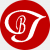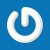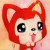﻿ php根据某一元素值进行数组过滤(筛选) - 小周博客,小周个人博客,程序猿小王子,技术博客,个人博客模板,php博客系统,设计模式,wzyl - 黑夜遮不住光亮

# php根据某一元素值进行数组过滤(筛选)

PHP按照某一元素值进行数组过滤，除了常用的遍历以外 我们可以用array_filter()函数实现，下面看示例

```\$arr = array(
0 => array('name' => '乔峰' , 'age' => 22,'trick' => '降龙十八掌'),
1 => array('name' => '杨过' , 'age' => 26,'trick' => '九阴真经'),
2 => array('name' => '张无忌','age' => 22,'trick' => '乾坤大挪移'),
);

//方式1 array_filter函数的第二个参数 直接写回调函数 function(\$variable){}

\$res = array_filter(\$arr,function(\$element){
return \$element['age'] == 22;  //只保留\$arr数组中的age元素为22的数组元素
});

var_dump(\$res); //输出结果如下：

array (size=2)
0 =>
array (size=3)
'name' => string '乔峰' (length=6)
'age' => int 22
'trick' => string '降龙十八掌' (length=15)
2 =>
array (size=3)
'name' => string '张无忌' (length=9)
'age' => int 22
'trick' => string '乾坤大挪移' (length=15)

//方式2 先定义一个函数 然后将函数传给array_filter()的第二个参数

function fun_callback(\$element)
{
//只保留array_filter()函数的第一个参数(第一个参数肯定是数组啦。)中的age元素为22的数组元素
return \$element['age'] == 22;
}

\$res = array_filter(\$arr,'fun_callback');

var_dump(\$res); //输出结果如下：

array (size=2)
0 =>
array (size=3)
'name' => string '乔峰' (length=6)
'age' => int 22
'trick' => string '降龙十八掌' (length=15)
2 =>
array (size=3)
'name' => string '张无忌' (length=9)
'age' => int 22
'trick' => string '乾坤大挪移' (length=15)

以上两种写法方式都是可以的。。 为了防止有些人看不懂。。就都写出来吧。。 更多细节请参看手册。。```

PS：array_filter(\$arr); 可以过滤空数组 无论是空数组 还是值是0 还是值是false 都会过滤掉(不过\$arr只能是一维数组，二维数组还没试验过)### 精彩评论

1.1楼

好牛，已测试

2016-02-28 12:04:01
2.2楼
naive

浏览器换了无数个都看不到密码

2017-02-23 04:06:13
•@naive 现在已被屏蔽。我再放上去，我的网站就被屏蔽咯

2017-02-24 01:46:37